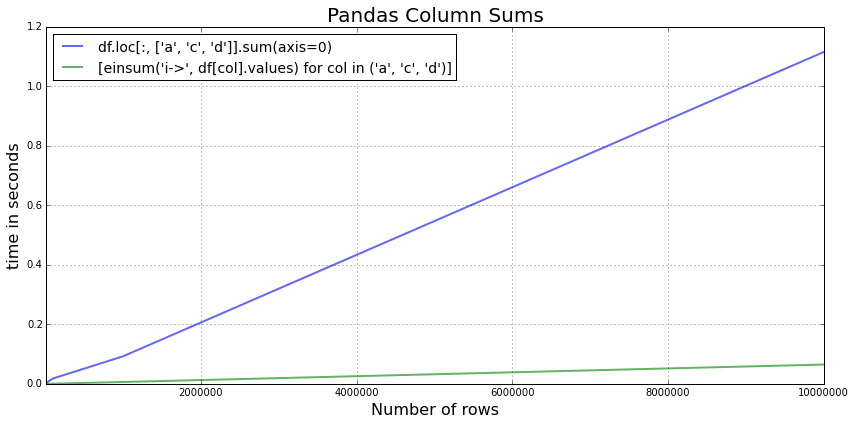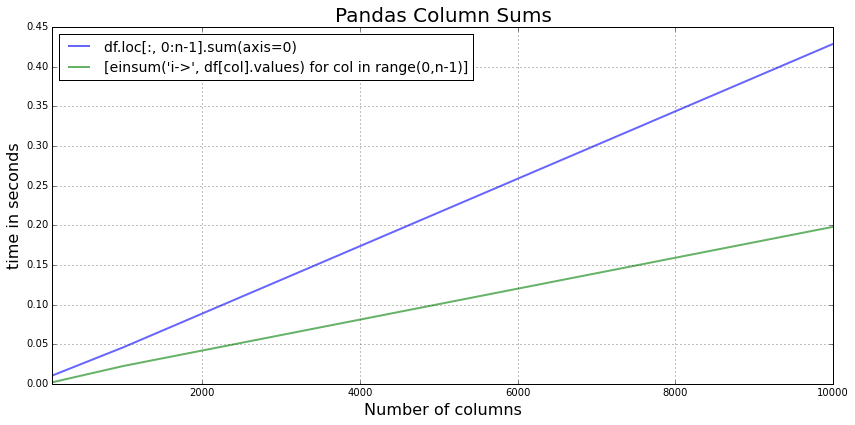In :
%load_ext watermark
%watermark -d -v -a 'Sebastian Raschka' -p numpy,pandas

The watermark extension is already loaded. To reload it, use:
Sebastian Raschka 24/12/2014

CPython 3.4.2
IPython 2.3.1

numpy 1.9.1
pandas 0.15.2


# 4 Simple Tricks To Speed up the Sum Calculation in Pandas¶

I wanted to improve the performance of some passages in my code a little bit and found that some simple tweaks can speed up the pandas section significantly. I thought that it might be one useful thing to share -- and no Cython or just-in-time compilation is required!

In my case, I had a large dataframe where I wanted to calculate the sum of specific columns for different combinations of rows (approx. 100,000,000 of them, that's why I was looking for ways to speed it up). Anyway, below is a simple toy DataFrame to explore the .sum() method a little bit.

In :
import pandas as pd
import numpy as np

df = pd.DataFrame()

for col in ('a', 'b', 'c', 'd'):
df[col] = pd.Series(range(1000), index=range(1000))

In :
df.tail()

Out:
a b c d
995 995 995 995 995
996 996 996 996 996
997 997 997 997 997
998 998 998 998 998
999 999 999 999 999

Let's assume we are interested in calculating the sum of column a, c, and d, which would look like this:

In :
df.loc[:, ['a', 'c', 'd']].sum(axis=0)

Out:
a    499500
c    499500
d    499500
dtype: int64

Now, the .loc method is probably the most "costliest" one for this kind of operation. Since we are only intersted in the resulting numbers (i.e., the column sums), there is no need to make a copy of the array. Anyway, let's use the method above as a reference for comparison:

In :
# 1
%timeit -n 1000 -r 5 df.loc[:, ['a', 'c', 'd']].sum(axis=0)

1000 loops, best of 5: 1.37 ms per loop


Although this is a rather small DataFrame (1000 x 4), let's see by how much we can speed it up using a different slicing method:

In :
# 2
%timeit -n 1000 -r 5 df[['a', 'c', 'd']].sum(axis=0)

1000 loops, best of 5: 986 µs per loop


Next, let us use the Numpy representation of the NDFrame via the .values attribue:

In :
# 3
%timeit -n 1000 -r 5 df[['a', 'c', 'd']].values.sum(axis=0)

1000 loops, best of 5: 687 µs per loop


While the speed improvements in #2 and #3 were not really a surprise, the next "trick" surprised me a little bit. Here, we are calculating the sum of each column separately rather than slicing the array.

In :
[df[col].values.sum(axis=0) for col in ('a', 'c', 'd')]

Out:
[499500, 499500, 499500]
In :
# 4
%timeit -n 1000 -r 5 [df[col].values.sum(axis=0) for col in ('a', 'c', 'd')]

1000 loops, best of 5: 64.4 µs per loop


In this case, this is an almost 10x improvement!

One more thing: Let's try the Einstein summation convention einsum.

In :
from numpy import einsum
[einsum('i->', df[col].values) for col in ('a', 'c', 'd')]

Out:
[499500, 499500, 499500]
In :
# 5
%timeit -n 1000 -r 5 [einsum('i->', df[col].values) for col in ('a', 'c', 'd')]

1000 loops, best of 5: 55.7 µs per loop


### Conclusion:¶

Using some simple tricks, the column sum calculation improved from 1370 to 57.7 µs per loop (approx. 25x faster!)

### What about larger DataFrames?¶

So, what does this trend look like for larger DataFrames?

In :
import timeit
import random
from numpy import einsum
import pandas as pd

def run_loc_sum(df):
return df.loc[:, ['a', 'c', 'd']].sum(axis=0)

def run_einsum(df):
return [einsum('i->', df[col].values) for col in ('a', 'c', 'd')]

orders = [10**i for i in range(4, 8)]
loc_res = []
einsum_res = []

for n in orders:

df = pd.DataFrame()
for col in ('a', 'b', 'c', 'd'):
df[col] = pd.Series(range(n), index=range(n))

print('n=%s (%s of %s)' %(n, orders.index(n)+1, len(orders)))

loc_res.append(min(timeit.Timer('run_loc_sum(df)' ,
'from __main__ import run_loc_sum, df').repeat(repeat=5, number=1)))

einsum_res.append(min(timeit.Timer('run_einsum(df)' ,
'from __main__ import run_einsum, df').repeat(repeat=5, number=1)))

print('finished')

n=10000 (1 of 4)
n=100000 (2 of 4)
n=1000000 (3 of 4)
n=10000000 (4 of 4)
finished

In :
%matplotlib inline

In :
from matplotlib import pyplot as plt

def plot_1():

fig = plt.figure(figsize=(12,6))

plt.plot(orders, loc_res,
label="df.loc[:, ['a', 'c', 'd']].sum(axis=0)",
lw=2, alpha=0.6)
plt.plot(orders,einsum_res,
label="[einsum('i->', df[col].values) for col in ('a', 'c', 'd')]",
lw=2, alpha=0.6)

plt.title('Pandas Column Sums', fontsize=20)
plt.xlim([min(orders), max(orders)])
plt.grid()

#plt.xscale('log')
plt.ticklabel_format(style='plain', axis='x')
plt.legend(loc='upper left', fontsize=14)
plt.xlabel('Number of rows', fontsize=16)
plt.ylabel('time in seconds', fontsize=16)

plt.tight_layout()
plt.show()

plot_1()It looks like that the benefit of calculating the sums separately for each column becomes even larger the more rows the DataFrame has.

Another question to ask: How does this scale if we have a growing number of columns?

In :
import timeit
import random
from numpy import einsum
import pandas as pd

def run_loc_sum(df, n):
return df.loc[:, 0:n-1].sum(axis=0)

def run_einsum(df, n):
return [einsum('i->', df[col].values) for col in range(0,n-1)]

orders = [10**i for i in range(2, 5)]
loc_res = []
einsum_res = []

for n in orders:

df = pd.DataFrame()
for col in range(n):
df[col] = pd.Series(range(1000), index=range(1000))

print('n=%s (%s of %s)' %(n, orders.index(n)+1, len(orders)))

loc_res.append(min(timeit.Timer('run_loc_sum(df, n)' ,
'from __main__ import run_loc_sum, df, n').repeat(repeat=5, number=1)))

einsum_res.append(min(timeit.Timer('run_einsum(df, n)' ,
'from __main__ import run_einsum, df, n').repeat(repeat=5, number=1)))

print('finished')

n=100 (1 of 3)
n=1000 (2 of 3)
n=10000 (3 of 3)
finished

In :
from matplotlib import pyplot as plt

def plot_2():

fig = plt.figure(figsize=(12,6))

plt.plot(orders, loc_res,
label="df.loc[:, 0:n-1].sum(axis=0)",
lw=2, alpha=0.6)
plt.plot(orders,einsum_res,
label="[einsum('i->', df[col].values) for col in range(0,n-1)]",
lw=2, alpha=0.6)

plt.title('Pandas Column Sums', fontsize=20)
plt.xlim([min(orders), max(orders)])
plt.grid()

#plt.xscale('log')
plt.ticklabel_format(style='plain', axis='x')
plt.legend(loc='upper left', fontsize=14)
plt.xlabel('Number of columns', fontsize=16)
plt.ylabel('time in seconds', fontsize=16)

plt.tight_layout()
plt.show()

plot_2()